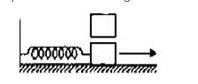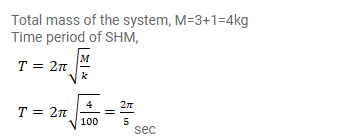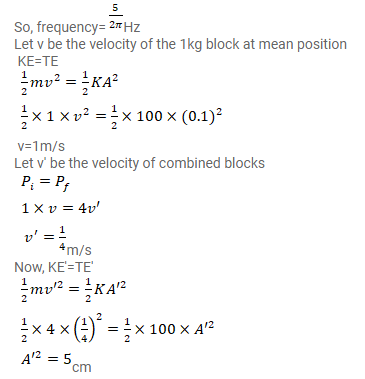# A 1kg block is executing simple harmonic motion of amplitude 0.1m

Question:

A $1 \mathrm{~kg}$ block is executing simple harmonic motion of amplitude $0.1 \mathrm{~m}$ on a smooth horizontal surface under the restoring force of a spring of spring constant $100 \mathrm{~N} / \mathrm{m}$. A block of mass $3 \mathrm{~kg}$ is gently placed on it at instant it passes through the mean position. Assuming that the two blocks moves together, find the frequency and amplitude of the motion.Solution: